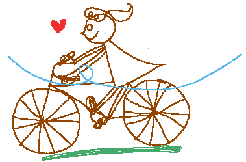WORKSHEET:PERCENTAGES 2 Worksheets Descargar PDF 1) Find the values: a) 75% of 2 005 b) 12% of 585 c) 25% of 2 370 d) 40% of 1 230 e) 20% of 54 f) 110% of 2 784 Solution 2) Find the value of X: a) 10% of X = 41 b) 100% of X = 3 287 c) 40% of X = 10 d) 60% of X = 180 e) 25% of X = 35 f) 95% of X = 288 Solution 3) Scott bought lunch for his friends at the Happy Hamburger. The total bill was \$24. Scott decided to leave a 15% tip for the waiter. How much was the tip?Solution 4) There are 540 students at Sunny Middle School. 432 students ride the bus to school. What percentage of the students do not ride the bus?Solution 5) 60 students participated in a survey about pets. 27 have a dog, 15 have a pet cat, and 18 students have no pets at all. What percentage of the students have a pet?Solution 6) Tim is buying a DVD player. The usual cost of the player is \$180. Today's discount is 20%. How much money will Tim save?Solution 7) Marys father said that he would pay 60% of the cost of a bicycle. The bicycle costs \$350. How much will Marys father pay?Solution 8) Greg had \$225 in his saving account. He spent 20% of his money on a present for his friend. How much money did Greg have left?Solution 9) Krystyl worked at the zoo. If 3 of her 15 baboons were sick. What percentage were sick?Solution 10) Matt worked as a produce manager for Walmark. If 35 people bought green peppers and this was 28% of the total customers, how many customers did he have?Solution